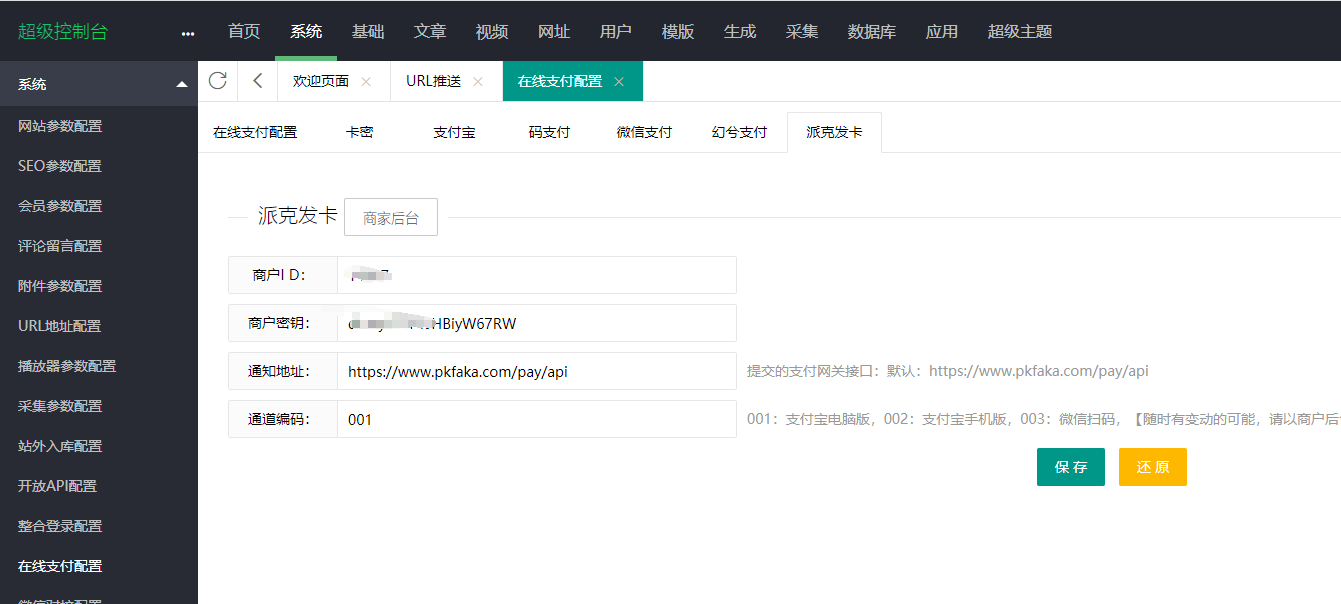## 应用介绍

•支付宝支付
•微信支付
•QQ钱包
•银联支付
•京东支付
•百度钱包
•Apple Pay
•蚂蚁花呗Q：怎么入驻自动发卡平台,成为商户?

A： 通过平台的账户注册功能，即可免费入驻自动发卡平台。

Q：怎么用平台销售虚拟商品？ 商户通过后台可以添加商品，每个商品自动发卡平台都会分配 一个购买链接，商户只要将这个链接发送给买家，买家付款后 平台自动发货，即可完成交易。

Q：选择一个安全的发卡网有多重要？

A：安全/隐私都值得重视，派克发卡网运用先进的安全技术保护用 户在账户中存储的个人信息、账户信息以及交易记录的安全。

Q：财务结算商户方式有那些？

A：支持支付宝、银行卡，微信结算。正常财务结算时间：最迟 午12点之前全部商户结算完毕。

Q：账户的金额满多少自动结算？

A：商户账户金额满50.00元，凌晨1点系统自动帮您提现，财务 于第二天12点前结算到您预留的账户，不满50.00元可以申请 手动提现，自动提现没有手续费。

```html

<script>

var codepay_type = '{maccms:foreach name=":explode(',',\$config.codepay.type)"}<option value ="{\$vo}">{if condition="\$vo==1"}支付宝二维码{elseif condition="\$vo==2"/}QQ钱包二维{elseif condition="\$vo==3"/}微信二维码{/if}</option>{/maccms:foreach}';

var zhapay_type ='{maccms:foreach name=":explode(',',\$config.zhapay.type)"}<option value ="{\$vo}">{if condition="\$vo==1"}微信{elseif condition="\$vo==2"/}支付宝{/if}</option> {/maccms:foreach}';

//修改过的开始

var pkfaka_type = '{maccms:foreach name=":explode(',',\$config.pkfaka.type)"}<option value ="{\$vo}">{if condition="\$vo==001"}支付宝电脑版{elseif condition="\$vo==002"/}支付宝手机版{elseif condition="\$vo==003"/}微信扫码{elseif condition="\$vo==004"/}微信H5{elseif condition="\$vo==005"/}微信公众支付{elseif condition="\$vo==006"/}QQ钱包{elseif condition="\$vo==007"/}京东支付{/if}</option>{/maccms:foreach}';

//修改过的结束

\$("#payment").change(function() {

\$('#paytype').html('');

if(\$("#payment").val()=="codepay" || \$("#payment").val()=="zhapay" || \$("#payment").val()=="Pkfaka"){

if(\$("#payment").val()=="codepay") {

\$('#paytype').html(codepay_type);

}

if(\$("#payment").val()=="zhapay") {

\$('#paytype').html(zhapay_type);

}

//修改过的开始

if(\$("#payment").val()=="Pkfaka") {

\$('#paytype').html(pkfaka_type);

}

//修改过的结束

\$("#paytype_box").slideDown();

}

else{

\$("#paytype_box").slideUp();

}

});

\$(".paytype").change(function() {

\$('#paytype').val( \$(this).val() );

});

</script>

```